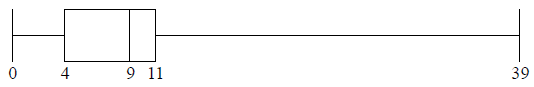# IB DP Maths Topic 4 : Statistics and probability-SL 4.3-Modal class -Question Bank SL Paper 1

## Question

The following box-and-whisker plot shows the number of text messages sent by students in a school on a particular day.Find the value of the interquartile range.


a.

One student sent k text messages, where k > 11 . Given that k is an outlier, find the least value of k.


b.

## Markscheme

recognizing Q1 or Q3 (seen anywhere)     (M1)

eg    4,11 , indicated on diagram

IQR = 7     A1 N2

[2 marks]

a.

recognizing the need to find 1.5 IQR     (M1)

eg   1.5 × IQR, 1.5 × 7

valid approach to find    (M1)

eg   10.5 + 11, 1.5 × IQR + Q3

21.5     (A1)

k = 22     A1 N3

Note: If no working shown, award N2 for an answer of 21.5.

[4 marks]

b.